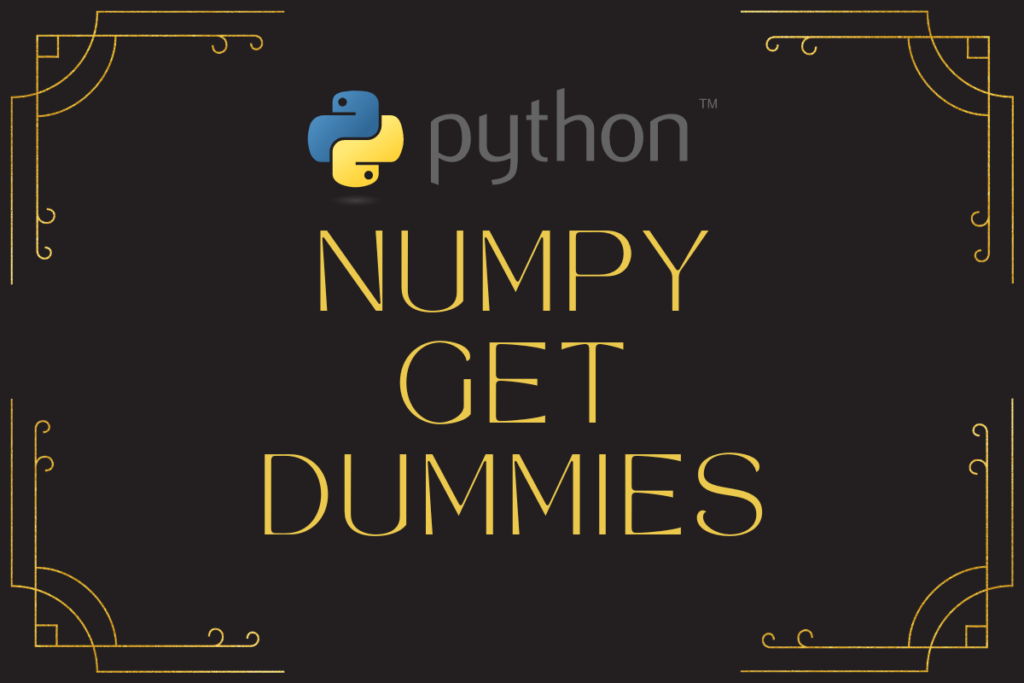# Mastering Pandas get_dummies(): A Guide for Python UsersData analytics has gone a long distance in quite a short time. With the technology advancing strength after strength in the field of computation and automation, new techniques have emerged to pump up the efficiency with which the data analysis is being carried out. This article shall focus on one such function from the pandas library of Python – the get_dummies( ) function. So, let us get started by importing this library using the below code.

```import pandas as pd
```

Thereafter, we shall explore further the get_dummies( ) function through each of the following sections.

• Why use a dummy variable?
• Syntax of the get_dummies( ) function
• Use cases for the get_dummies( ) function

## Why use a dummy variable?

Those familiar with machine learning know, how numerical things can get. Numbers are always better to analyze than case-sensitive alphabets; bring in the tildes & all goes swoosh! So, the dummy variables might be a savior in that case.

They work like a charm when it comes to machine learning algorithms such as regression which strictly deal with numbers. Have no belief? Try feeding in some textual data into your linear regression and witness the montage of errors being thrown at, the very moment the code is run!

## Syntax of the get_dummies() function

Dummy variables ease the treacherous task of data cleaning by assigning a numerical value to the categorical data of the given dataframe. Following is the syntax of the get_dummies( ) function detailing the fundamental constituents required for its proper functioning.

```pandas.get_dummies(data, prefix=None, prefix_sep=’_’, dummy_na=False, columns=None, sparse=False, drop_first=False, dtype=None)
```

where,

• data – Categorical dataframe that is to be converted into dummy variables
• prefix – An optional component set to ‘None’ by default and is used to assign column names to the dummy variable dataframe
• prefix_sep – An optional component set to ‘_’ by default and is used to differentiate the categorical entry from the column name in the dummy variable dataframe
• dummy_na – An optional component set to ‘False’ by default and is used to add a column to indicate the positions where there are zeros in every column of the dummy variable dataframe
• columns – An optional component set to ‘None’ by default and is used to encode the column names in the input categorical dataframe before conversion into dummy variables
• sparse – An optional component set to ‘False’ by default and is set to ‘True’ if the dummy encoded columns are to be backed by a sparse array rather than a numpy array
• drop_first – An optional component set to ‘False’ by default and is set to ‘True’ if the first level from the input categorical data is to be removed while converting to dummy variables
• dtype – An optional component set to ‘None’ by default and is used to specify the data type for the new columns of dummy variables

## Use cases for the get_dummies() function

In this section, we shall demonstrate the use of a handful of components within the get_dummies( ) function with the following dataframe.

```import numpy as np
Input = pd.DataFrame({"ID":[1002, 3201, 4031, 2078, 5897],
"Region":["Africa","Europe","Asia","Africa", np.nan]})
print(Input)
```

We shall use only the Region column from the above dataframe for conversion into dummy variables.

```Region = Input.Region
print(Region)
```

Once done, let us try running it through the get_dummies( ) function with its default setting.

```pd.get_dummies(Region)
```

Now let us deploy some of the components within the get_dummies( ) function to do the following,

• Assign a prefix ‘option’ with ‘-‘ as a separator
• Create an additional column to indicate the locations where values are not available
• Remove the first level of categorical data
• Return all dummy variables as ‘float’ data type

All the above-listed requirements when translated into a code become the ones given below.

```Res = pd.get_dummies(Region, prefix='option', prefix_sep="-", dummy_na=True, drop_first=True, dtype=float)
print(Res)
```